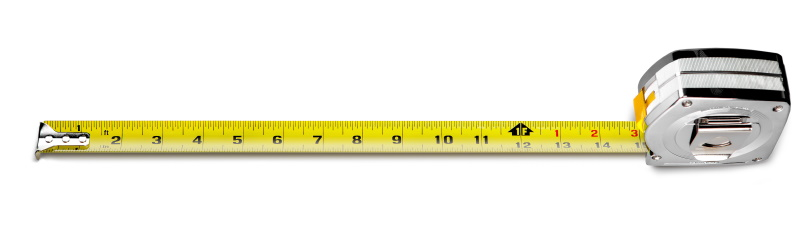# From tape measures to tensorsThis post will start with a motivating example, looking at measuring a room in inches and in feet. Then we will segue into a discussion of contravariance and covariance in the simplest setting. Then we will discuss contravariant and covariant tensors more generally.

## Using a tape measure

In my previous post, I explained why it doesn’t matter if a tape measure is perfectly straight when measuring a long distance. In a nutshell, if you want to measure x, but instead you measure the hypotenuse of a triangle with sides x and y, where y is much smaller than x, the difference is approximately y²/2x. The error is nowhere as big as y.

In that post I gave the example of measuring a wall that is 10 feet long, and measuring to a point 4 inches up the adjacent wall rather than measuring to the corner. The error is about 1/15 of an inch.

Now suppose we’re off by more, measuring 12 inches up the other wall. Now that we have an even foot, we can switch over to feet and work with smaller, simpler numbers. Now we have x = 10 and y = 1. So the error is approximately 1/20.

Before, we were working in inches. We had x = 120, y = 4, and error 1/15. Does that mean our error is now smaller? That can’t be. If the short leg of our triangle is longer, 12 inches rather than 4 inches, our error should go up, not down.

Of course the resolution is that our error was 1/15 of an inch in the first example, and 1/20 of a foot in the second example. If we were to redo our second example in inches, we’d get error 12²/240 = 12/20, i.e. we’d convert 1/20 of a foot to 12/20 of an inch.

## Change of units and contravariance

Now here’s where tensors come in. Notice that when we use a larger unit of measurement, a foot instead of an inch, we get a smaller numerical value for error. Trivial, right? If you first measure a distance in meters, you’ll get larger numbers if you switch to centimeters, but smaller numbers if you switch to light years.

But this simple observation is an example of a deeper pattern. Measurements of this kind are contravariant, meaning that our numerical values change in the opposite direction as our units of measurement.

A velocity vector is contravariant because if you use smaller units of length, you get larger numerical values of velocity, and vice versa. Under a change of units, velocity changes in the opposite direction of the units.

A gradient vector is covariant because if you use smaller units of length, a function will vary less per unit length. Gradients change in the same direction as your units.

The discussion so far has been informal and limited to a very special change of coordinates. It’s not just the direction of change that matters, that results change monotonically with units, but that they increase or decrease by the exact same proportion. And the kinds of coordinate changes we usually have in mind are not changing from inches to feet but rather changing from rectangular coordinates to polar coordinates.

## More general and more formal

Suppose you have some function T described by coordinates denoted by x‘s with superscripts. Put bars on top of everything to denote a new representation of T with respect to new coordinates. If T is a contravariant vector we have,and if T is a covariant vector we haveIn the equations above there is an implicit summation over the repeated index r, using the so-called Einstein summation convention.

The examples at the end of the previous section are the canonical examples: tangent vectors are contravariant and gradients are covariant.

If the xs without bars are measured in inches and the xs with bars are measured in feet, the partial derivative of an x bar with respect to the corresponding x is 1/12, because a unit change in inches causes a change of 1/12 in feet.

Vectors are a special case of tensors, called 1-tensors. Higher order tensors satisfy analogous rules. A 2-tensor is contravariant ifand covariant ifEven more generally you can have tensors of any order, and they can be contravariant in some components and covariant in others.

## Backing up

For more on tensors, you may want to read a five-part series of blog posts I wrote starting with What is a tensor?. The word “tensor” is used in several related but different ways. The view of tensors given here, as things that transform a certain way under changes of coordinates, is discussed in the fourth post in that series.

## 3 thoughts on “From tape measures to tensors”

1. Velocity has two units, distance and time, and while velocity gets smaller as the units of length get larger, it gets larger as the units of time get larger. How does this relate to covariance and contravariance? Does only the numerator count?

2. Is there meaning to T_rs vs T↑rs, or is that a typo?

3. @Tomas:

There is a meaning to the subscripts and superscripts: indices for contravariant components go up, indices for covariate components go down.